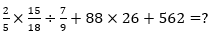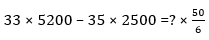Dear Aspirants,

Quantitative Aptitude Quiz For SBI PO/Clerk Prelims

Numerical Ability or Quantitative Aptitude Section has given heebie-jeebies to the aspirants when they appear for a banking examination. As the level of every other section is only getting complex and convoluted, there is no doubt that this section, too, makes your blood run cold. The questions asked in this section are calculative and very time-consuming. But once dealt with proper strategy, speed, and accuracy, this section can get you the maximum marks in the examination. Following is the Quantitative Aptitude quiz to help you practice with the best of latest pattern questions.

Q1. The ratio between sides of a square & an equilateral triangle is 5 : 3. The area of triangle is 36√3 m², Find the perimeter of square.
80 m
96 m
100 m
64 m
120 m

Q2. The ratio of volumes of two cylinders A and B is 7 : 10. If the ratio between heights of cylinders A and B is 8 : 7 respectively then what is the ratio between curved surface area of cylinders ?
3 : √5
5: √2
2 : √5
4 : √5
2 : 2√5

Q3. A metal cube having side of 8 m is melted and rerated into 64 small cubes. Find the surface area of single small cube.
36 m²
24 m²
20 m²
25 m²
32 m²

Q4. A goat is bound at one corner of a square field of side 20 m. If the length of rope is 7/10 time of that of side of field then find area grazed by goat .
54 m²
124 m²
154 m²
254 m²
196 m²

Q5. Find the maximum length of a rod which can be placed inside a room of size 8m × 9m × 12m.
14 m
15 m
19 m
17 m
21 m

Directions (6-10): The following pie chart shows the percentage distribution of scholarship money obtained by six students of a university in Rs. 500 and Rs. 1000 notes. The table shows the ratio of no. of Rs.500 and Rs.1000 notes obtained by the students. Study the graphs carefully and answer the related questions.
Total money of scholarship distributed to the six students = Rs.7.5 lakhs
Note: In entire money there are in only Rs.500 and Rs.1000 notes.
Q6. Total   money obtained by Ravi in the form of Rs 500 notes is what percent of total money obtained by Raju in the form of Rs.1000 notes?

Q6. Total money obtained by Ravi in the form of Rs 500 notes is what percent of total money obtained by Raju in the form of Rs.1000 notes?
120%
125%
115%
130%
140%
Solution:
Let no. of 500rupee and 1000rupee notes obtained by Ravi are 3x and x respectively.

∴ Amount obtained by Ravi in the form of 500rupee notes = 3 × 100 × 500 = Rs 1,50,000
Similarly, amount obtained by Raju in the form of 1000rupee notes
let no. of 500rupee and 1000rupee notes obtained by Raju are y and 2y respectively.

Q7. What is the ratio of money obtained by Riya & Ravi together to that by Raghu and Raja together?
43 : 70
40 : 71
71 : 40
70 : 43
83 : 40

Q8. What is the average no. of Rs.500 notes obtained by Rita & Riya together?
70
65
60
50
55

Q9. Total no. of Rs.1000 notes obtained by Raja is how much percent more or less than the total no. of Rs.1000 notes obtained by Riya?
100/3%
37/3%
31/3%
50/3%
25/2%

Q10. What is the difference between total no. of Rs.1000 notes obtained by Ravi and Raju together and Rs.500 notes obtained by Ravi and Raju together?
130
140
120
80
110

Directions (11 - 15): What value will come in place of (?) in the following questions?

Q11. 55.55 – 46.46 + 90.90 + 88.88 = 521 - ?
331
332.13
335.42
401
231.42

Q12.Q13.19
22
25
23
27

Q14.10052
14528
12212
10092
12090

Q15.1099
1199
1000
1212
1010

You May also like to Read: